David Mumford did receive earlier this year the 2007 AMS Leroy P. Steele Prize for Mathematical Exposition. The jury honors Mumford for “his beautiful expository accounts of a host of aspects of algebraic geometry”. Not surprisingly, the first work they mention are his mimeographed notes of the first 3 chapters of a course in algebraic geometry, usually called “Mumford’s red book” because the notes were wrapped in a red cover. In 1988, the notes were reprinted by Springer-Verlag. Unfortnately, the only red they preserved was in the title.

The AMS describes the importance of the red book as follows. “This is one of the few books that attempt to convey in pictures some of the highly abstract notions that arise in the field of algebraic geometry. In his response upon receiving the prize, Mumford recalled that some of his drawings from The Red Book were included in a collection called Five Centuries of French Mathematics. This seemed fitting, he noted: “After all, it was the French who started impressionist painting and isn’t this just an impressionist scheme for rendering geometry?””

These days it is perfectly possible to get a good grasp on difficult concepts from algebraic geometry by reading blogs, watching YouTube or plugging in equations to sophisticated math-programs. In the early seventies though, if you wanted to know what Grothendieck’s scheme-revolution was all about you had no choice but to wade through the EGA’s and SGA’s and they were notorious for being extremely user-unfriendly regarding illustrations…

So the few depictions of schemes available, drawn by people sufficiently fluent in Grothendieck’s new geometric language had no less than treasure-map-cult-status and were studied in minute detail. Mumford’s red book was a gold mine for such treasure maps. Here’s my favorite one, scanned from the original mimeographed notes (it looks somewhat tidier in the Springer-version)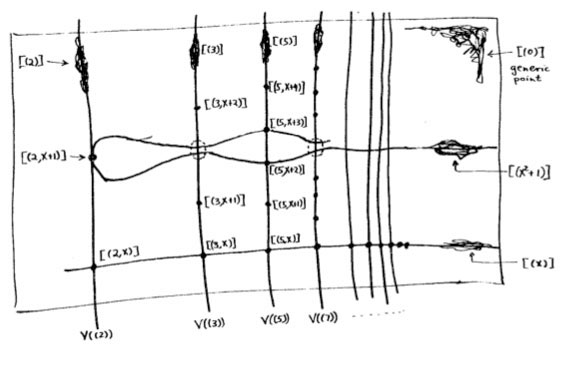It is the first depiction of $\mathbf{spec}(\mathbb{Z}[x])$, the affine scheme of the ring $\mathbb{Z}[x]$ of all integral polynomials. Mumford calls it the”arithmetic surface” as the picture resembles the one he made before of the affine scheme $\mathbf{spec}(\mathbb{C}[x,y])$ corresponding to the two-dimensional complex affine space $\mathbb{A}^2_{\mathbb{C}}$. Mumford adds that the arithmetic surface is ‘the first example which has a real mixing of arithmetic and geometric properties’.

Let’s have a closer look at the treasure map. It introduces some new signs which must have looked exotic at the time, but have since become standard tools to depict algebraic schemes.

For starters, recall that the underlying topological space of $\mathbf{spec}(\mathbb{Z}[x])$ is the set of all prime ideals of the integral polynomial ring $\mathbb{Z}[x]$, so the map tries to list them all as well as their inclusions/intersections.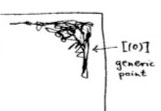The doodle in the right upper corner depicts the ‘generic point’ of the scheme. That is, the geometric object corresponding to the prime ideal $~(0)$ (note that $\mathbb{Z}[x]$ is an integral domain). Because the zero ideal is contained in any other prime ideal, the algebraic/geometric mantra (“inclusions reverse when shifting between algebra and geometry”) asserts that the gemetric object corresponding to $~(0)$ should contain all other geometric objects of the arithmetic plane, so it is just the whole plane! Clearly, it is rather senseless to depict this fact by coloring the whole plane black as then we wouldn’t be able to see the finer objects. Mumford’s solution to this is to draw a hairy ball, which in this case, is sufficiently thick to include fragments going in every possible direction. In general, one should read these doodles as saying that the geometric object represented by this doodle contains all other objects seen elsewhere in the picture if the hairy-ball-doodle includes stuff pointing in the direction of the smaller object. So, in the case of the object corresponding to $~(0)$, the doodle has pointers going everywhere, saying that the geometric object contains all other objects depicted.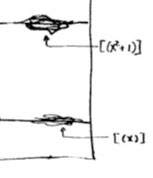Let’s move over to the doodles in the lower right-hand corner. They represent the geometric object corresponding to principal prime ideals of the form $~(p(x))$, where $p(x)$ in an irreducible polynomial over the integers, that is, a polynomial which we cannot write as the product of two smaller integral polynomials. The objects corresponding to such prime ideals should be thought of as ‘horizontal’ curves in the plane.

The doodles depicted correspond to the prime ideal $~(x)$, containing all polynomials divisible by $x$ so when we divide it out we get, as expected, a domain $\mathbb{Z}[x]/(x) \simeq \mathbb{Z}$, and the one corresponding to the ideal $~(x^2+1)$, containing all polynomials divisible by $x^2+1$, which can be proved to be a prime ideals of $\mathbb{Z}[x]$ by observing that after factoring out we get $\mathbb{Z}[x]/(x^2+1) \simeq \mathbb{Z}[i]$, the domain of all Gaussian integers $\mathbb{Z}[i]$. The corresponding doodles (the ‘generic points’ of the curvy-objects) have a predominant horizontal component as they have the express the fact that they depict horizontal curves in the plane. It is no coincidence that the doodle of $~(x^2+1)$ is somewhat bulkier than the one of $~(x)$ as the later one must only depict the fact that all points lying on the straight line to its left belong to it, whereas the former one must claim inclusion of all points lying on the ‘quadric’ it determines.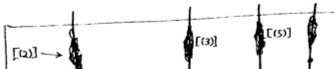Apart from these ‘horizontal’ curves, there are also ‘vertical’ lines corresponding to the principal prime ideals $~(p)$, containing the polynomials, all of which coefficients are divisible by the prime number $p$. These are indeed prime ideals of $\mathbb{Z}[x]$, because their quotients are
$\mathbb{Z}[x]/(p) \simeq (\mathbb{Z}/p\mathbb{Z})[x]$ are domains, being the ring of polynomials over the finite field $\mathbb{Z}/p\mathbb{Z} = \mathbb{F}_p$. The doodles corresponding to these prime ideals have a predominant vertical component (depicting the ‘vertical’ lines) and have a uniform thickness for all prime numbers $p$ as each of them only has to claim ownership of the points lying on the vertical line under them.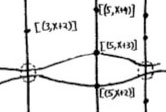Right! So far we managed to depict the zero prime ideal (the whole plane) and the principal prime ideals of $\mathbb{Z}[x]$ (the horizontal curves and the vertical lines). Remains to depict the maximal ideals. These are all known to be of the form
$\mathfrak{m} = (p,f(x))$
where $p$ is a prime number and $f(x)$ is an irreducible integral polynomial, which remains irreducible when reduced modulo $p$ (that is, if we reduce all coefficients of the integral polynomial $f(x)$ modulo $p$ we obtain an irreducible polynomial in $~\mathbb{F}_p[x]$). By the algebra/geometry mantra mentioned before, the geometric object corresponding to such a maximal ideal can be seen as the ‘intersection’ of an horizontal curve (the object corresponding to the principal prime ideal $~(f(x))$) and a vertical line (corresponding to the prime ideal $~(p)$). Because maximal ideals do not contain any other prime ideals, there is no reason to have a doodle associated to $\mathfrak{m}$ and we can just depict it by a “point” in the plane, more precisely the intersection-point of the horizontal curve with the vertical line determined by $\mathfrak{m}=(p,f(x))$. Still, Mumford’s treasure map doesn’t treat all “points” equally. For example, the point corresponding to the maximal ideal $\mathfrak{m}_1 = (3,x+2)$ is depicted by a solid dot $\mathbf{.}$, whereas the point corresponding to the maximal ideal $\mathfrak{m}_2 = (3,x^2+1)$ is represented by a fatter point $\circ$. The distinction between the two ‘points’ becomes evident when we look at the corresponding quotients (which we know have to be fields). We have

$\mathbb{Z}[x]/\mathfrak{m}_1 = \mathbb{Z}[x]/(3,x+2)=(\mathbb{Z}/3\mathbb{Z})[x]/(x+2) = \mathbb{Z}/3\mathbb{Z} = \mathbb{F}_3$ whereas $\mathbb{Z}[x]/\mathfrak{m}_2 = \mathbb{Z}[x]/(3,x^2+1) = \mathbb{Z}/3\mathbb{Z}[x]/(x^2+1) = \mathbb{F}_3[x]/(x^2+1) = \mathbb{F}_{3^2}$

because the polynomial $x^2+1$ remains irreducible over $\mathbb{F}_3$, the quotient $\mathbb{F}_3[x]/(x^2+1)$ is no longer the prime-field $\mathbb{F}_3$ but a quadratic field extension of it, that is, the finite field consisting of 9 elements $\mathbb{F}_{3^2}$. That is, we represent the ‘points’ lying on the vertical line corresponding to the principal prime ideal $~(p)$ by a solid dot . when their quotient (aka residue field is the prime field $~\mathbb{F}_p$, by a bigger point $\circ$ when its residue field is the finite field $~\mathbb{F}_{p^2}$, by an even fatter point $\bigcirc$ when its residue field is $~\mathbb{F}_{p^3}$ and so on, and on. The larger the residue field, the ‘fatter’ the corresponding point.

In fact, the ‘fat-point’ signs in Mumford’s treasure map are an attempt to depict the fact that an affine scheme contains a lot more information than just the set of all prime ideals. In fact, an affine scheme determines (and is determined by) a “functor of points”. That is, to every field (or even every commutative ring) the affine scheme assigns the set of its ‘points’ defined over that field (or ring). For example, the $~\mathbb{F}_p$-points of $\mathbf{spec}(\mathbb{Z}[x])$ are the solid . points on the vertical line $~(p)$, the $~\mathbb{F}_{p^2}$-points of $\mathbf{spec}(\mathbb{Z}[x])$ are the solid . points and the slightly bigger $\circ$ points on that vertical line, and so on.

This concludes our first attempt to decypher Mumford’s drawing, but if we delve a bit deeper, we are bound to find even more treasures… (to be continued).

Published in featured